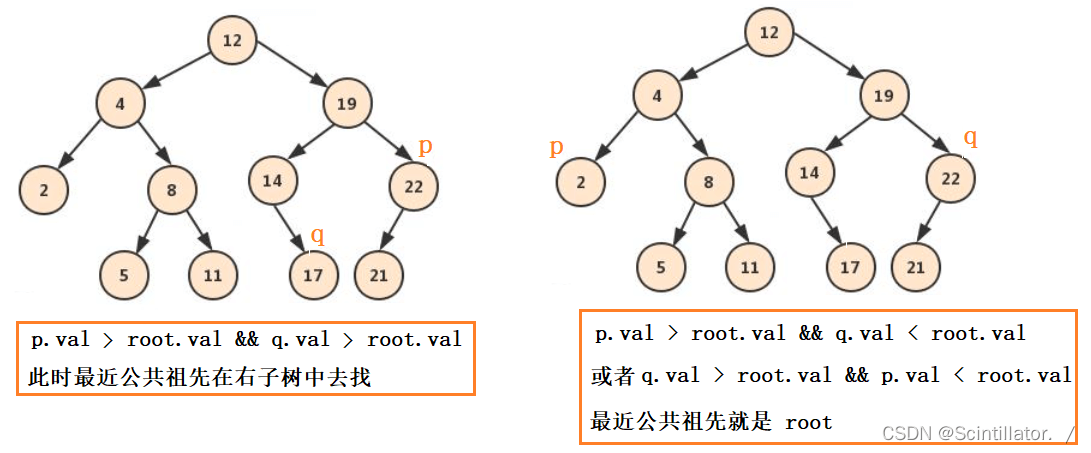﻿ Java 讲解两种找二叉树的最近公共祖先的方法_java_脚本之家
java# Java 讲解两种找二叉树的最近公共祖先的方法

## 思路一：先假设这棵树是二叉搜索树```public TreeNode lowestCommonAncestor(TreeNode root, TreeNode p, TreeNode q) {
if(root == null) return null;

// p 为当前树的根节点
if(p == root) return p;
// q 为当前树的根节点
if(q == root) return q;

// 去左子树中找
TreeNode left = lowestCommonAncestor(root.left,p,q);
// 去右子树中找
TreeNode right = lowestCommonAncestor(root.right,p,q);

// 左边右边都找到了
if(left != null && right != null) {
return root;
}
// 左边找到了，右边没找到
if(left != null) {
return left;
}
if(right != null) {
return right;
}
return null;
}
```

## 思路二：假设该树是用孩子双亲表示法```// 用于找节点的路径
public boolean getPath(TreeNode root, TreeNode node, Stack<TreeNode> stack) {
if(root == null || node == null) {
return false;
}
// 将当前节点放入栈中
stack.push(root);

if(root.val == node.val) {
return true;// 找到了
}
// 当前节点没找到，去左子树找
boolean flag = getPath(root.left,node,stack);
// 左子树中找到了，直接返回
if(flag) {
return true;
}
// 左子树没找到，去右子树找
flag = getPath(root.right,node,stack);
// 右子树中找到了，直接返回
if(flag) {
return true;
}

// 左右子树都没找到，弹出节点
stack.pop();
return false;

}
public TreeNode lowestCommonAncestor(TreeNode root, TreeNode p, TreeNode q) {
if(root == null) {
return null;
}
Stack<TreeNode> stackp = new Stack<>();
Stack<TreeNode> stackq = new Stack<>();

// 分别得到  p q 的路径
getPath(root,p,stackp);
getPath(root,q,stackq);

int sizep = stackp.size();
int sizeq = stackq.size();

if(sizep > sizeq) {
int size = sizep - sizeq;
// 弹出元素直至两栈中元素个数相等
while(size > 0) {
stackp.pop();
size--;
}
}else {
int size = sizeq - sizep;
// 弹出元素直至两栈中元素个数相等
while(size > 0) {
stackq.pop();
size--;
}
}

// 一起弹出，直到找到第一个相同的元素
while(!stackp.isEmpty() && !stackq.isEmpty()) {
if(stackp.peek() == stackq.peek()) {
// 找到了，就返回该节点
return stackq.pop();
}else {
stackp.pop();
stackq.pop();
}
}
// 没找到，返回 null
return null;
}
```